# SpacePhasor

Physical transformation: three phase <-> space phasors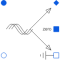# Information

This information is part of the Modelica Standard Library maintained by the Modelica Association.

Physical transformation of voltages and currents: three phases <-> space phasors:
x[k] = X0 + {cos(-(k - 1)/m*2*pi),-sin(-(k - 1)/m*2*pi)}*X[Re,Im]
and vice versa:
X0 = sum(x[k])/m
X[Re,Im] = sum(2/m*{cos((k - 1)/m*2*pi),sin((k - 1)/m*2*pi)}*x[k])
were x designates three phase values, X[Re,Im] designates the space phasor and X0 designates the zero sequence system.
Physical transformation means that both voltages and currents are transformed in both directions.
Zero-sequence voltage and current are present at pin zero. An additional zero-sequence impedance could be connected between pin zero and pin ground.

# Parameters (1)

turnsRatio Value: 1 Type: Real Description: Turns ratio

# Connectors (5)

plug_p plug_n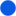Type: PositivePlug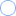Type: NegativePlug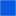Type: PositivePin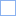Type: NegativePin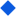Type: SpacePhasor

# Used in Components (2)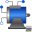AIM_SlipRing Modelica.Electrical.Machines.BasicMachines.AsynchronousInductionMachines Asynchronous induction machine with slipring rotor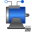PartialBasicInductionMachine Modelica.Electrical.Machines.Interfaces Partial model for induction machine